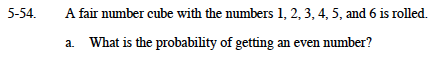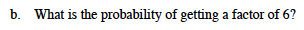### Home > MC1 > Chapter 5 > Lesson 5.2.2 > Problem5-54

5-54.How many even numbers are there on the cube? How many possibilities are there for what can be rolled?

There are 3 even numbers (2, 4, 6) and 6 possibilities, so the probability of rolling an even number is

$\frac{3}{6}\text{ or }\frac{1}{2}$Follow the same steps as outlined in part (a).

1, 2, 3, and 6 are all factors of six.